# GATE CS 2016 Mock Solutions

GATE CS 2017 Mock Test

GATE CS 2016 Mock Solutions
(Q1 to Q5 carry 1 mark each)

QUESTION 1 : The candidate from our constituency was attacked during his __________ for the MP elections.

A) canvas
B) canvass
C) canvus
D) canvaass

Correct – B

canvas – a type of cloth
canvass – a campaign or survey

QUESTION 2 : Which of the following has the closest meaning to the sentence below :
She is an __________ writer. Everyone in the industry respects her.

A) imminent
B) emminent
C) eminent
D) aminent

Correct – C

imminent – about to happen, to take place very soon
eminent – remarkable, distinguished

QUESTION 3 : Which of the following pairs are not related :

A) bold – timid
B) clumsy – graceful
C) hinder – help
D) irritate – nettle

Correct – D

Irritate and Nettle are synonyms. Both mean to excite a feeling of anger. Rest all are antonyms.

QUESTION 4 : Select the most appropriate option that would solve the problem.

Problem – What is the profit made by the shopkeeper?
Statements:
(I) The shopkeeper sold 200 articles at Rs.10 each.
(II) The profit per article is same as the cost price per article.

A) I alone is sufficient while II alone is not sufficient
B) II alone is sufficient while I alone is not sufficient
C) I and II are together sufficient, but neither alone
D) I and II together are not sufficient

Correct – C

From (I), selling price per article = Rs. 10
From (II), cost price per article = profit per article
Now, selling price = cost price + profit
So, cost price per article = profit per article = Rs. 5
Total 200 articles are sold.
Thus, total profit = Rs. 1000
Therefore, both (I) and (II) are together sufficient, but neither alone.

QUESTION 5 : What is the probability of getting atmost two tails when an unbiased coin is tossed thrice?

A) 7 / 8
B) 1 / 8
C) 3 / 8
D) 5 / 8

Correct – A

Sample space = {HHH, HHT, HTH, THH, HTT, THT, TTH, TTT}
Desired options are HHH, HHT, HTH, THH, HTT, THT, TTH.
Thus, P(atmost two tails) = 7/8

(Q6 to Q10 carry 2 marks each)

QUESTION 6 : Select the option closest to the meaning of the idiom – Skeleton crew

A) A group of stupid people
B) A group of highly skilled people
C) Minimum number of people required to keep a job running
D) A group of lazy people

Correct – C

Skeleton crew means the least number of employees required to keep a work running

QUESTION 7 : For the given statement, select the assumption(s) that follow:

Statement – A large number of people from city S are moving to city T owing to extremely high temperature.
Assumption :
(I) City T has lower temperature than city S.
(II) City T has adequate resources to meet this shift.

A) I follows
B) II follows
C) Both I and II follow
D) None follows

Correct – A

I is true as city T must be having lower temperature than city S. That is the reason why the people of city S have decided to move to city T.

QUESTION 8 : A bag contains 5 balls out of which some or maybe all are black. 2 balls are drawn from the bag and both are found to be black. What is the probability that all balls in the bag are black?
_________

Correct – 0.5

Since 2 black balls were drawn, the number of black balls may be either of 2,3,4,5.
Let A2 = bag contains 2 black balls
A3 = bag contains 3 black balls
A4 = bag contains 4 black balls
A5 = bag contains 5 black balls
E = 2 black balls are drawn

P(E / A2) = 2C2 / 5C2 = 1/10
P(E / A3) = 3C2 / 5C2 = 3/10
P(E / A4) = 4C2 / 5C2 = 3/5
P(E / A5) = 5C2 / 5C2 = 1

Since the number of black balls is unknown, all events A2, A3, A4, A5 are equally likely.
So, P(A2) = P(A3) = P(A4) = P(A5) = 1/4

By Bayes’ theorem,
P(A5 / E) = {P(A5) X P(E / A5) } / [{P(A2) X P(E / A2) } + {P(A3) X P(E / A3) } + {P(A4) X P(E / A4) } + {P(A5) X P(E / A5) }]

P(A5 / E) = {1/4 X 1} / [{1/4 X 1/10} + {1/4 X 3/10} + {1/4 X 3/5} + {1/4 X 1}]

P(A5 / E) = 1/2 = 0.5

QUESTION 9 : Answer the question on the basis of the given table.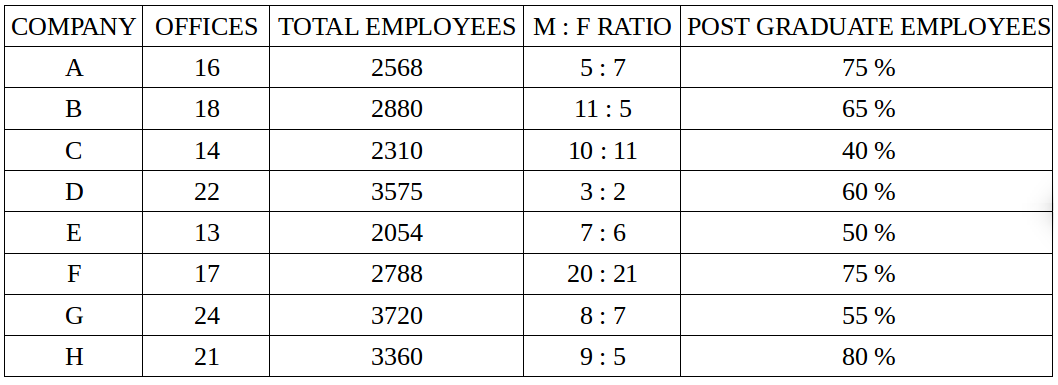If the number of male post–graduate employees in company H is 1800, what percent of female employees in that particular company is NOT post–graduate?

A) 76
B) 74
C) 26
D) 24

Correct – C

Number of post graduate employees in company H = 80% of 3360 = 2688
Number of male post–graduate employees in company H = 1800
Therefore, number of female post–graduate employees in company H = 2688 – 1800 = 888
Also, Total female employees in company H = (5 / 14) * 3360 = 1200
So, number of female non post–graduate employees in company H = 1200 – 888 = 312
Hence, required percentage = (312 / 1200) * 100 = 26 %.

QUESTION 10 : Answer the question on the basis of the given table.Which company has the highest number of employees per office?

A) Company F
B) Company H
C) Company B
D) Company C

Correct – D

F = 2788 / 17 = 164
H = 3360 / 21 = 160
B = 2880 / 18 = 160
C = 2310 / 14 = 165

(Q11 to Q35 carry 1 mark each)

QUESTION 11 : Given h(z) = (z+2)2 + 3(z+2) – 8, determine two functions f(z) and g(z) which when composed together as f(g(z)) will generate h(z).

A) f(z) = z2 + 3z – 8, g(z) = z
B) f(z) = z2 + 3z + 8, g(z) = z+2
C) f(z) = z2 + 3z – 8, g(z) = z+2
D) f(z) = z2 + 3z + 8, g(z) = z–2

Correct – C

From option C, f(g(z)) = f(z+2) = (z+2)2 + 3(z+2) – 8 = h(z)

QUESTION 12 : What is the value of limx–>0 (cos x – 1) / x

A) e
B) 0
C) 1
D) None of These

Correct – B

There is a property in limits that limx–>0 (cos x – 1) / x = 0.
http://www.mathportal.org/formulas/limits_and_derivatives_formulas.pdf

QUESTION 13 : Match the best case complexity :

```(P) Selection Sort            (X) O ( n log(n) )
(Q) Insertion Sort            (Y) O ( n2 )
(R) Heap Sort                (Z) O ( n )
```

A) P – X, Q – Z, R – Y
B) P – Y, Q – Z, R – X
C) P – Z, Q – Y, R – X
D) All have best case complexity O ( log(n) )

Correct – B

Selection sort has a complexity O ( n2 ) in every case.
Insertion sort has the best case when the input is already sorted. Every insert requires constant time.
Heap sort has a complexity O ( n log(n) ) in every case.

QUESTION 14 : Consider the below Pseudo code written in C style

```bool fun(int arr[], int n, int X)
{
if (X == 0)     return true;
if (n == 0 && X != 0)     return false;

if (arr[n–1]*arr[n–1] > X)     return fun(arr, n–1, X);

// Here "|| "indicates logical or
return fun(arr, n–1, X) || fun(arr, n–1, X – arrr[n–1]*arr[n–1]);
}
```

Which of the following is true about above code.
A) Time Complexity of fun() is O(2n) and it requires O(n) extra space
B) Time Complexity of fun() is O(2n) and it requires O(n2) extra space
C) Time Complexity of fun() is O(n2) and it requires O(n) extra space
D) Time Complexity of fun() is O(n2) and it requires O(n2) extra space

Correct – A

T(n) = 2 T(n–1) + c, which is O(2n).
Since there can be atmost O(n) elements in function call stack, extra space required is O(n).

QUESTION 15 : Consider the below Pseudo code written in C style

```bool fun(int arr[], int n, int X)
{
if (X == 0)     return true;
if (n == 0 && X != 0)     return false;

if (arr[n–1]*arr[n–1] > X)     return fun(arr, n–1, X);

// Here "|| "indicates logical or
return fun(arr, n–1, X) || fun(arr, n–1, X – arrr[n–1]*arr[n–1]);
}
```

Which of the following is true about the function in the above code.

A) Returns true if there are two subsequences of arr[] with product as X.
B) Returns false if there are two subsequences of arr[] with product as X.
C) Returns true if there is a subsequences with sum of squares equal to X.
D) Returns false if there is a subsequences with sum of squares equal to X.

Correct – C

The function represents code for a variation of subset sum problem.
Here we find if there is a subsequences with sum of squares of elements equal to X or not.

QUESTION 16 : Consider the following tree. How many nodes will have the same position regardless of the fact whether the tree is traversed in DFS or BFS order? (Consider that we select children from right to left starting from the rightmost child)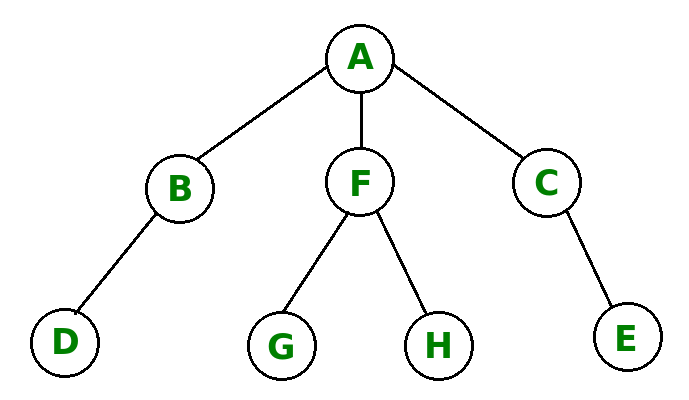________

Correct – 3

DFS – ACEFHGBD
BFS – ACFBEHGD
So, A,C and D have the same position.
Thus, 3 is the required answer.

QUESTION 17 : The following numbers are inserted into an empty binary search tree in the given order: 10, 1, 3, 5, 15, 12, 16, 4, 2. What is the height of the binary search tree?
(Here height is defined as number of edges on longest path from root to a leaf)

A) 2
B) 3
C) 4
D) 5

Correct – C
The height of a binary search tree is the maximum distance of a leaf node from the root.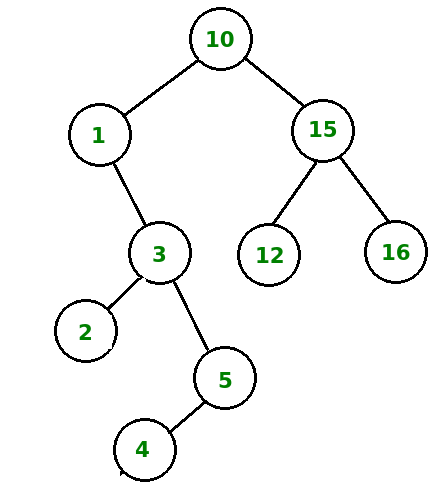QUESTION 18 : Which of the following is true about the given grammar

```S –> iCtSE | a
E –> eS | eps
C –> b
```

(NOTE : Here, ‘eps’ represesnts epsilon)

A) The grammar is LL(1)
B) The grammar is not LL(1)
C) The grammar is incomplete
D) None of the above

Correct – B

FIRST (S) = {i, a}
FIRST (E) = {e, eps}
FIRST (C) = {b}

When we make the LL(1) parse table, we get a conflict in E row.
Thus, the given grammar is not LL(1).

QUESTION 19 : Which of the following is equivalent to :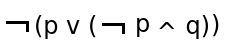```(i)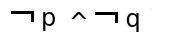(ii)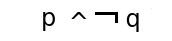(iii)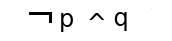(iv)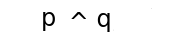```

Correct – A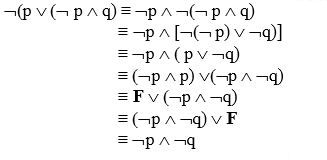http://www.csitschools.com/

QUESTION 20 : Which of the following sets will have same number of elements in their power set?
Set 1 = {1, 2, 3, 4, 5, 6}
Set 2 = {{1, 2}, {3, 4}, {5, 6}}
Set 3 = {1, 2, 3, 4, {5, 6}}
Set 4 = {{1, 2, 3}, {4, 5, 6}}

A) Set 1, Set 2, Set 4
B) Set 1, Set 2
C) All have same number of elements in their power set
D) No two sets have same number of elements in their power set

Correct – D

No. of elements in Set 1 = 6. So, No. of elements in the power set of Set 1 = 26 = 64
No. of elements in Set 2 = 3. So, No. of elements in the power set of Set 1 = 23 = 8
No. of elements in Set 3 = 5. So, No. of elements in the power set of Set 1 = 25 = 32
No. of elements in Set 4 = 2. So, No. of elements in the power set of Set 1 = 22 = 4

QUESTION 21 : Consider a 4 – bit Johnson Counter initialized to ‘0000’. What is the number of clock cycles required for the counter to reset to ‘0000’?
_____

Correct – 8

The Johnson counter resets the input, i.e., reaches the initial input in ‘2n’ clock cycles, where ‘n’ is the number of bits. So, for 4 – bit counter, the counter will reset in 2 X 4 = 8 clock cycles.

QUESTION 22 : Consider register reference instructions. Which one of the following is true:

A) First 12 bits (0 – 11) specify register operation, last 4 bits (12 – 15) are always 1111
B) First 11 bits (0 – 10) specify register operation, last 5 bits (11 – 15) are always 01111
C) First 12 bits (0 – 11) specify register operation, last 4 bits (12 – 15) are always 1110
D) First 4 bits (0 – 3) are always 0111, last 12 bits (4 – 15) specify register operation

Correct – C

In register reference instructions, the first 12 bits (0 – 11) specify the register operation.
The next three bits are 111 and specify opcode and the last bit of the instruction is mode which is 0.
Therefore, last 4 bits are always 1110.

QUESTION 23 : Which class of ports does the port number 48151 belongs?

A) The ‘well – known’ ports
B) Registered ports
C) Private ports
D) Free ports

Correct – B

Port numbers 1 – 1023 belong to the ‘well – known’ ports.
Port numbers 1024 – 49151 belong to Registered ports
Port numbers 49152 – 65535 belong to Private ports.

QUESTION 24 : Consider the fragment offset field in the IPv4 header. What is its maximum value in bytes ?
____

Correct – 65528

The length of fragment offset field is 13 bits. Also, fragment offset is measured in 8 – byte blocks.
So, maximum offset is (213 – 1) X 8 = 65528 bytes.

QUESTION 25 : Match the protocols with the port numbers.

```(P) HTTPS            (W) 110
(Q) SNMP            (X) 161
(R) SMB                    (Y) 443
(S) POP3            (Z) 445
```

A) P – W, Q – Z, R – Y, S – X
B) P – X, Q – W, R – Z, S – Y
C) P – Y, Q – X, R – W, S – Z
D) P – Y, Q – X, R – Z, S – W

Correct – D

HTTPS – 443
SNMP – 161
SMB – 445
POP3 – 110

QUESTION 26 : Consider below the regular expressions over alphabet {0, 1, 2}

(i) (0 + 01 + 012))*
(ii) 0*(0 + 1)*(0+1+2)*
(iii) 0*(ε + 01)*(Φ + 012)*
(iv) 0* + (01)* + (012)*

Which of the above regular expressions represent same language as 0*(01)*(012)*

(A) i, ii and iii
(B) ii and iv
(C) iii
(D) iv

Correct – C

Making a DFA is all that is required to get the correct answer.

QUESTION 27 : Assuming a 16 – bit address space with 12 logical pages. What is the size of each page ?

A) 1K
B) 2K
C) 4K
D) None of These

Correct – C

It takes 4 bits to reference 12 logical pages (24 = 16, so maximum 16 pages can be referenced in 4 – bits). Now, we are left with 16 – 4 = 12 bits, which can be used for pages.
So, page size = 212 = 4K

QUESTION 28 : What is the output of the following C program :

```#include “stdio.h”
int main()
{
int i = –2, j = 2, k = 1, m;
m = ++i? ––k && ++i && ++j : ++k && ––i && ++j ;
printf("%d, %d, %d, %dn", i, j, k, m);
return 0;
}
```

A) –2, 3, 2, 1
B) –1, 2, 0, 0
C) 0, 3, 0, 0
D) –1, 2, 1, 0

Correct – B

For calculating ‘m’, any non – zero value is considered TRUE. In conditional operator, ++i makes i = –1, which makes it go to the TRUE case of the conditional operator. Now, ––k will return 0 and since there is AND after that, it will not be calculated any further as False && False or False && True will always return False.
So, i = –1, j = 2, k = 0, m = 0.

QUESTION 29 : Consider a table STUDENTS and the following two SQL queries.
Query 1 – DELETE FROM STUDENTS;
Query 2 – TRUNCATE TABLE STUDENTS;

Which of the following is TRUE :

A) Query 2 will fire any associated triggers whereas Query 1 will not
B) Both the queries are exactly same
C) Both the queries remove all rows from the table and this cannot be undone
D) Only Query 1 requires some other operation to make the removal permanent

Correct – D

Both the queries will remove all the rows from the table. But, query 1 can be undone by using rollback whereas query 2 is permanent. Also, DELETE fires all the associated triggers whereas TRUNCATE does not.

QUESTION 30 :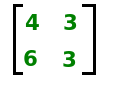What is the sum of all the elements of the L and U matrices as obtained in the L U decomposition?

A) 16
B) 10
C) 9
D) 6

Correct – C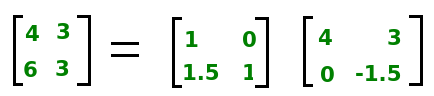So, the sum of all elements is 9.

QUESTION 31 : What is the output of the following C program :

```#include “stdio.h”
int main()
{
float i, j;
i = 5 * 5 / 21;
j = 5 * 5 / 21.0;
printf("%f * %f",i,j);
return 0;
}
```

A) 0.000000 * 0.000000
B) 1.000000 * 1.190476
C) 0.000000 * 1.190476
D) 1.000000 * 1.000000

Correct – B

Computation for ‘i’ would be done using integer arithmetic whereas for ‘j’, it would be done using double or floating point arithmetic. Also, the precedence of ‘*’ and ‘/’ would be same and the expression would be calculated from Left to Right.

QUESTION 32 : Consider following three function written in C style :

```
void fun1(int n)
{
int i;
for (i=1; i<n*n*n; i=i*2)
{
// Do some O(1) works
}
}

void fun2(int n)
{
int i;
for (i=1; i<10000; i=i*2)
{
// Do some O(1) works
}
}

void fun3(int n)
{
int i;
for (i=1; i<n*n*n; i=i+2)
{
// Do some O(1) works
}
}
```

Which of the following is true :

i) Time Complexity of fun1() is Θ(n3)
ii) Time Complexity of fun3() is Θ(n3)
iii) Time Complexity of fun2() is Θ(1)
iv) Time Complexity of fun1() is O(log n)
v) Time Complexity of fun3() is Θ(n2)

A) ii, iii and iv
B) i, ii and iii
C) iii, iv and v
D) i, iii and v

Correct – A

Time Complexity of fun1() – Θ(log (n3)) = Θ(log n)
Time Complexity of fun2() – O(1)
Time Complexity of fun3() – Θ(n3)

QUESTION 33 : Consider a network path with three links.
Link 1 – Speed : 10 Gbps, Propagation Delay : 40ms
Link 2 – Speed : 2 Gbps, Propagation Delay : 5ms
Link 3 – Speed : 2 Gbps, Propagation Delay : 10ms
Assume processing delay to be zero.
A data packet of 500 KB is sent from a source to a destination via the path : link 1 – link 3 – link 2.
What is the total delay in transmitting the packet?

A) 54.90 ms
B) 50.20 ms
C) 55.55 ms
D) 59.40 ms

Correct – D

Total delay = Propagation delay + Transmission Delay + Queuing delay + Processing delay
Total delay = 40ms + 10ms + 5ms + 500KB X (1/10Gbps + 1/2Gbps + 1/2Gbps)
Total delay = 59.40 ms
NOTE : Here, ‘B’ is bytes (in data) and ‘b’ is bits (in speed)

QUESTION 34 : Consider below statements about Heap Data Structure

i) Time complexity of making a Min Heap from n unsorted elements is O(n)
ii) Heap is preferred over Linked list for implementation of Doubly Ended Queue.
iii) Heap is preferred over Binary Search Tree for implementation of Priority Queue.
iv) To heapify a node in Heap, all subtrees of the node must be heapified.
v) If we use Singly Linked List instead of array for implementation Heap, then time complexity of build heap increases.

Which of the above are true?

A) Only i, ii, iii and v
B) Only i, iii and iv
C) Only i, iii, iv and v
D) Only i and iv

Correct – C

(i) is true (Refer https://www.geeksforgeeks.org/g-fact-85/)
(ii) is false
(iii) is true (Refer https://www.geeksforgeeks.org/why-is-binary-heap-preferred-over-bst-for-priority-queue/)
(iv) is true (Refer https://www.geeksforgeeks.org/data-structure-gq/heap-gq/)
(v) Build Heap requires random access to find first internal node and to find parents of nodes which is not possible in (1) time with linked list.

QUESTION 35 : Consider below program

```Line 1 : #include “stdio.h”
Line 2 : int main(void)
Line 3 : {
Line 4 : int a = 1, 2, 3;
Line 5 : printf("%d", a);
Line 6 : return 0;
Line 7 : }
```

Which of the following is true about the program:

A) Compiler Error in Line 4
B) Linker Error in Line 4
C) No Error, it prints 1
D) Linker Error in Line 5

Correct – A

Comma is used either as a separator or an operator. Here, it is used in line 4 as a separator rather than operator. It should be written as :

```int a;
a = 1, 2, 3;
```

(Q36 to Q65 carry 2 marks each)

QUESTION 36 : Assume CSMA/CD protocol. Find the least frame length in bytes for a 2 Mbps bit rate and 1.5km long network where propagation delay is 4.25 nano seconds per metre.

______

Correct – 3.1875

Minimum frame size for CSMA/CD = 2 X Propagation delay
Minimum frame size = 2 X Propagation Delay per metre X Length of the network X Bit Rate
Minimum frame size = 2 X 4.25 X 10– 9 X 1.5 X 103 X 2 X 106
So, minimum frame size = 25.50/8 = 3.1875 bytes

QUESTION 37 : Which of the following has the longest range ?

A) WiFi : 802.11n
B) WiFi : 802.11g
C) WiFi : 802.11b
D) WiFi : 802.11a

Correct – A

WiFi : 802.11n has the highest range among the other given choices.

QUESTION 38 : Which of the following follow commutative law but not associative law?

A) AND
B) OR
C) XOR
D) NAND

Correct – D

AND, OR, XOR and XNOR follow associative law.

QUESTION 39 : Solve upto 2 places after the decimal :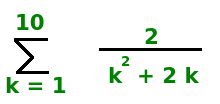______

Correct – 1.32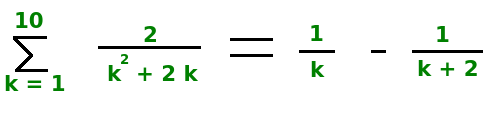On opening the summation, we would be left with (1) + (1/2) – (1/11) – (1/12), which is equal to 175/132. Taking upto 2 places after the decimal, we get 1.32 as our answer.

QUESTION 40 : Consider a function f(A,B,C,D) defined by the summation of the terms 1, 5, 6, 7, 11, 12, 13, 15. What is the value of function ‘f’ in the SOP form?

A) A’ C’ D + A B C’ + A’ B C + A C D
B) A’ C’ D’ + A’ B C’ + A’ B C + A C D
C) A’ C’ D + A’ B C’ + A’ B C’ + A’ C’ D
D) A’ C’ D + A’ B C’ + A’ B’ C + A’ C’ D

Correct – A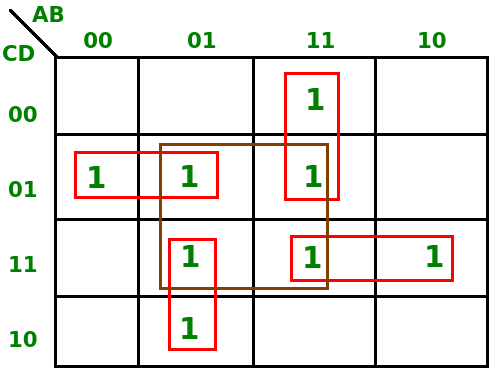QUESTION 41 : Consider the recurrence relation T(n) = T(n–1) + T(n/2) + n.

Which of the following is a good tight upper bound on T(n)

(A) Θ(n2)
(B) Θ(n2 log n)
(C) Θ(2 (log n)2)
(D) Θ(n (log n)2)

Correct – C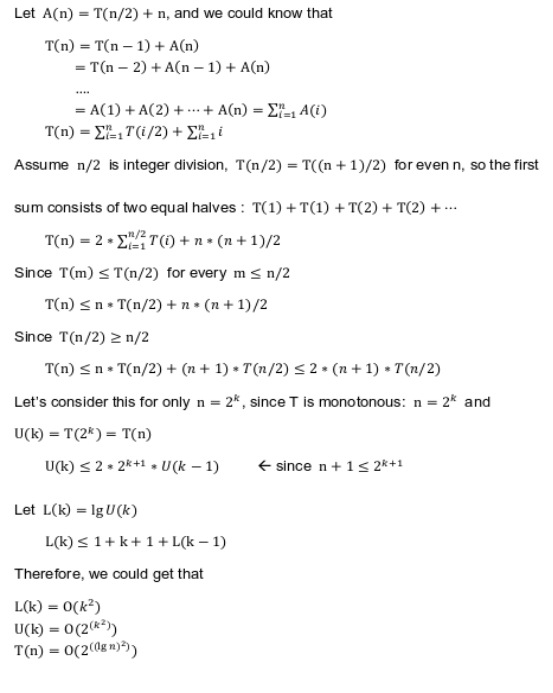http://hscc.cs.nthu.edu.tw/~sheujp/lecture_note/13algorithm/ch4%20solution.pdf

QUESTION 42 : WiFi is :

A) Simplex
B) Full Duplex
C) Half Duplex
D) None of the above

Correct – C

WiFi is half duplex.

QUESTION 43 : Consider the grammar given below:

```            S → AS | b
A → SA| a
```

The grammar is :

(i) LL(1)

(ii)LR(0)

(iii)LR(1)

(A) (i) & (iii) are correct
(B) (i) & (ii) are correct
(C) All are correct
(D) All are incorrect

Correct – D

The Grammar is ambiguous. For a string “abab” two derivations are possible and no ambiguous grammar can be LR.
Derivation 1 : S → AS → SAS → ASAS → aSAS → abAS → abaS → abab
Derivation 2 : S → AS → aS → aAS → aSAS → abAS → abaS → abab

QUESTION 44 : Consider the automaton X over the alphabet {x,y,z}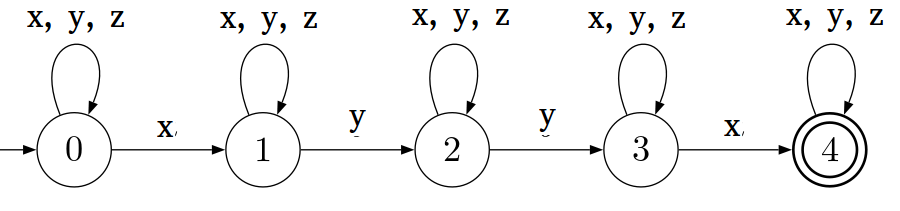Which of the below statements are true about the diagram:
(i) ε ∈ L(X)
(ii) The Automaton is a DFA.
(iii) yxzxyzx ∈ L(X)
(iv) yyxxzyxyzxz ∈ L(X)
(v) The Language accepted by X contains words that contain at least one x, y, y and x in that order.

(A) i, iii, iv and v
(B) ii, iv, v
(C) iii, v
(D) iv, v

Correct – D

(i) is false: empty strings can’t be accepted
(ii) is false: as we can go to multiple next states from state 1 and symbol x.
(iii) is false: There is no path that can lead to final state for yxzxyzx
(iv) is true : yyxxzyxyzxz is acceptable by NFA
(v) is true : We move to final state only when we have seen x, y, y and x in that order

QUESTION 45 : Which of the following statements are true?
S1 : A language accepted by Non – Deterministic PDA can also be accepted by Deterministic PDA.
S2 : A language accepted by Non – Deterministic FA can also be accepted by Deterministic FA.
S3 : A language accepted by Non – Deterministic Turing Machine can also be accepted by Deterministic Turing Machine.

A) S1, S2
B) S2, S3
C) S1, S3
D) only S2

Correct – B

QUESTION 46 : Consider three processes P1, P2 and P3 arriving at 0ms, 4ms and 10ms respectively and have burst times 8ms, 4ms and 1ms respectively. Which of the following will have the highest average waiting time ?

A) First Come First Serve (FCFS)
B) Pre – emptive Shortest Job First
C) Non Pre – emptive Shortest Job First
D) Round Robin with quantum 1

Correct – D

Round Robin with time quantum 1 will have the highest average waiting time of 3ms. Rest all will have average waiting time less than 3ms.

QUESTION 47 : Consider the following statements :
(i) T flip flop can be designed using D flip flop
(ii) JK flip flop can be designed using D flip flop
(iii) JK flip flop can not be designed using T flip flop

Which of the above are True ?

A) Only (i)
B) Only (iii)
C) (i) and (ii)
D) All are True

Correct – C

It is possible to design JK flip flop using T flip flop.
T = J Qn‘ + K Qn

QUESTION 48 : Consider an instruction pipeline with four stages (S1, S2, S3 and S4) each with combinational circuit only. The pipeline registers are required between each stage and at the end of the last stage. Delays for the stages and for the pipeline registers are as given in the figure: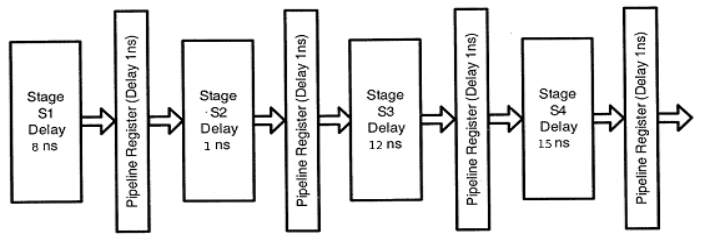What is the approximate speed up of the pipeline in steady state under ideal conditions when compared to the corresponding non-pipeline implementation?

_____________

Correct – 2.25

Time without pipeline = 8 + 1 + 12 + 15 ns = 36 ns
Time with pipeline = 15 + 1 ns = 16 ns
Speedup = 36/16 = 2.25

QUESTION 49 : Assume two relations R1(X,Y) with Primary key Y and 1000 tuples and R2(Y,Z) with primary key YZ and 2000 tuples and it is given that R2. Y is foreign key to R1.Y
What will be maximum and minimum number of tuples in natural join of R and S?

A) Max 2000 and Min 1000
B) Max 2000 and Min 0
C) Both 2000
D) Max 1000 and Min 0

Correct – C

QUESTION 50 : Select the option that will give highest number of page faults for the sequence – 7, 0, 1, 2, 0, 3, 0, 4, 2, 3, 0, 3, 2, 1, 2, 0, 1, 7, 0, 1.

A) Optimal Page Replacement Algorithm with 3 frames
B) LRU Page Replacement Algorithm with 3 frames
C) Optimal Page Replacement Algorithm with 4 frames
D) LRU Page Replacement Algorithm with 4 frames

Correct – B

Optimal Page Replacement Algorithm with 3 frames – 9 page faults
Optimal Page Replacement Algorithm with 4 frames – 8 page faults
LRU Page Replacement Algorithm with 3 frames – 12 page faults
LRU Page Replacement Algorithm with 4 frames – 8 page faults

QUESTION 51 : Solve :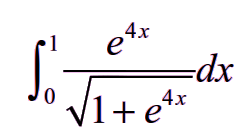Round off the answer to the nearest integer.

______

Correct – 3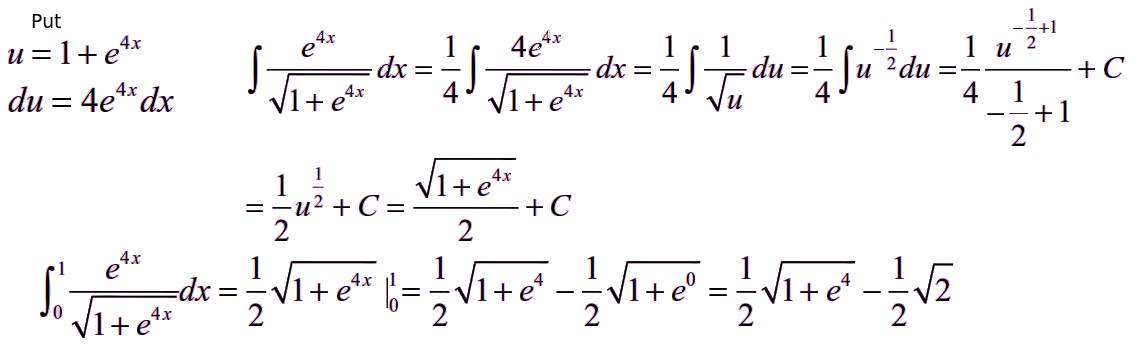QUESTION 52 : What is mod(product of eigen values) for the matrix :

```1    2    -2
1    1     1
1    3    -1
```

Correct – 4

Th eigen values of the matrix are 1, 2 and -2, the product of which is -4.
Taking mod, the answer is 4.

QUESTION 53 : Consider the following query on a relation REL(X,Y,Z) with candidate keys X and Y.

Q1: select count(*) from (select X,Y from REL)R1 NATURAL JOIN (select Y,Z from REL)R2;
Q2: select count(*) from (select X,Y from REL)R1 NATURAL JOIN (select X,Z from REL)R2;
Q3: select count(*) from (select X,Z from REL)R1 NATURAL JOIN (select Y,Z from REL)R2;

The result of which queries will be equivalent ?

A) Q1, Q2
B) Q2, Q3
C) Q1, Q3
D) All will have the same result

Correct – A

QUESTION 54 : Predict the output of the C program:

```#include “stdio.h”
void fun(int *p)
{
static int q = 10;
p = &q;
}

int main()
{
int r = 123;
int *p = &r;
fun(p);
printf("%d", *p);
getchar();
return 0;
}
```

Correct – 123

Inside fun(), q is a copy of the pointer p. So if we change q to point something else then p remains unaffected.

QUESTION 55 : Consider a Binary Tree where the allowed height difference between left and right children of every node is at most one. What are the maximum and minimum possible heights of such a Binary Tree ?

(A) Minimum Height : floor (log n) and Maximum Height : approximately 1.44 log n

(B) Minimum Height : floor (log n) and Maximum Height : ceil (log n)

(C) Minimum Height : ceil (log n) and Maximum Height : floor (n/2)

(D) Minimum Height : approximately 1.44 log n and Maximum Height : floor (n/2)

Correct – A

The binary tree as per the question would be an AVL Tree.
Height of AVL would be minimum when tree is complete like Heap, which is floor(log n).
Height would be maximum when differences between heights is exactly one for every internal node, which is approximately 1.44 log n.

QUESTION 56 : What is the solution of the following recurrence relation :

T(n) = T(n/4) + T(n/2) + n3
T(1) = c
T(0) = 0

A) O (n4)
B) O (n3)
C) O (n3 log n)
D) O (n2 log n)

Correct – B

We can solve it by drawing a recursion tree similar to https://www.geeksforgeeks.org/algorithms-analysis-of-algorithms-question-1/

QUESTION 57 : Consider a system generating 20 bit frames and connected through a shared 20kbps channel. Find throughput in percent if slotted ALOHA is used and frame rate is 1000 fps.

________________

Correct – 37

Frame size = L = 20 bits
Rate = R = 20kbps
Transmission time,T = L/R = 1 X 10-3 s
Throughput, S = G e-G, where G= Number of frames per T
So, G = 1.
Therefore, S = e-1 = 37 % approximately

QUESTION 58 : Consider a graph with ‘n’ nodes such that 1 node is master node and all other are slave nodes such that the master is connected to all slaves but no two slaves are connected. What is the chromatic number for such graph?

A) n
B) n-1
C) n+1
D) None of these

Correct – D

Lets say that we give color RED to master. Since slaves are connected to the master, no slave can be given RED color but since all of them are mutually unconnected, they all can be given same color, say GREEN. So, we need only two colors.

QUESTION 59 : Match the correct choices :

```(P) Array                (W) Average case access – O(n)
(Q) Binary Search Tree            (X) Worst case access – O(n)
(R) Doubly Linked List            (Y) Worst case access – O(1)
(S) B Tree                (Z) Average case access – O(log n)
```

A) P – W, Q – X, R – Y, S – Z
B) P – X, Q – W, R – Y, S – Z
C) P – Y, Q – X, R – W, S – Z
D) P – Z, Q – X, R – W, S – Y

Correct – C

QUESTION 60 : In the Lexical Analysis, regular expression can be used to model

A) the structures of lexemes with fixed length identifier excluded
B) the structure of tokens
C) the structure of tokens but not lexemes
D) the structure of lexemes with variable length identifier included

Correct – D

The structure of all lexemes(of any length) in the program can be verified & described by a Regular Grammar, and a Regular Grammar can be described using a Regular Expression.

QUESTION 61 : Match the correct choices :

```(P) Transport Layer            (W) IP Address
(Q) Network Layer            (X) MAC Address
```

A) P – W, Q – X, R – Y
B) P – X, Q – W, R – Y
C) P – Y, Q – W, R – X
D) None of the above is correct

Correct – C

QUESTION 62 : A relation R has no composite candidate keys. Which of the following is always true for relation R?

(A) R is in 2NF
(B) R is in 3NF
(C) R is in BCNF
(D) Can’t be predicted

Correct – A

A relation without composite keys will always satisfy the conditions for a relation to be in 2NF.

QUESTION 63 : Consider a frame 1101011011 and a generator polynomial 10011. What is the number of 1’s in the transmitted frame?
________

Correct – 10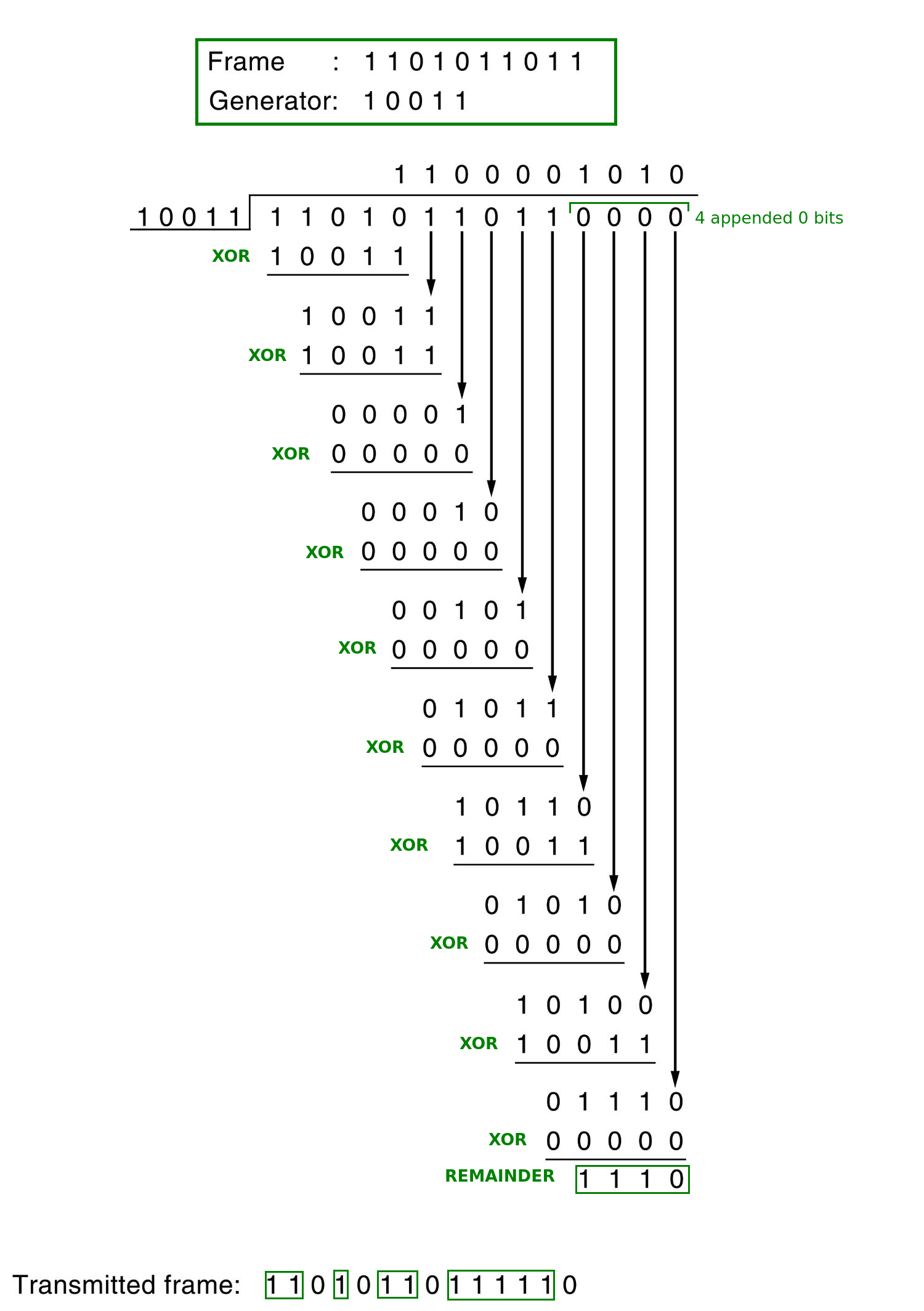So, the number of 1’s in the transmitted frame is 10.

QUESTION 64 : Consider a symmetric key cryptography system with 15 users. What is the number of keys required for this system?

________

Correct – 105

Number of keys = (15 X 14) / 2 = 105

QUESTION 65 : Consider following forwarding table in router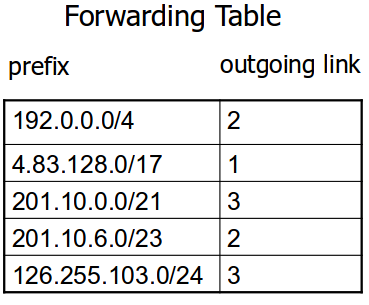An incoming packet with IP address 201.10.7.17 is forwarded to outgoing link :

A) 3
B) 2
C) 2 and 3
D) 1, 2 and 3

Correct – B

Routers use Longest Prefix Matching rule. The rule is to find the entry in table which has the longest prefix matching with incoming packet’s destination IP, and forward the packet to corresponding next hop.

Longest Prefix Matching in Routers

http://inst.eecs.berkeley.edu/~ee122/fa09/notes/08-Forwarding-Transportx6.pdf